In this blog entry, I will be attempting to explain merge sort to the best of my abilities. I will try to cover everything from the basics of what merge sort is to its functioning and I will also lay out its pros and cons as a sorting algorithm.

## What is Merge Sort?

Merge sort is a recursive sorting algorithm based on the divide and conquer technique. It is one of the most respected algorithms in data structures. One interesting thing to note about merge sort is that all its time complexities i.e Best, Worst and Average case is:

→Ο(n log n)

## How does Merge Sort works?

It recursively divides the given array into two sub-arrays, then calls itself on the two halves, this goes on till each element is an individual subarray. It then compares the adjacent elements and then merges them creating a sorted array.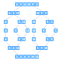source: https://www.programiz.com/dsa/merge-sort

If we to sort an array A. We can sort a sub-section of this array starting at index x and ending at index y, denoted as A[x..y].

Divide
We can find a q as the mid-point of x and y, then we can create two subarrays of A[x..y] as A[x..q] and A[q+1, y].

Conquer
In this step, we try to sort both the sub-arrays A[x..q] and A[q+1, y]. Until we reach the base case we further divide the subarrays into more subarrays and try to sort them.

Combine
If the base case is satisfied we get two sorted subarrays A[x..q]and A[q+1, y] for array A[x..y], we compare the sorted subarrays and combine them to create a sorted A[x…y].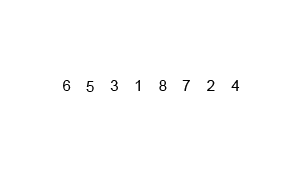source: https://en.wikipedia.org/wiki/Merge_sort#/media/File:Merge-sort-example-300px.gif

Working Steps:

1. From the input array, the middle element is chosen.
2. Two new sub-arrays of equal size are created.
3. Elements from the 0th index to the middle index is filled in the first array and elements from the middle+1 index to the end of the original array are filled into the second subarray.
4. This goes on for all the sub-arrays until the base case is met which is the size of the newly created subarray ≤1.
5. At that point, the merge function is called.
6. The merge function compares the adjacent sub-arrays and finally returns the sorted array.

MergeSort Function Layout:

`MergeSort(arr[], l,  r)If r > l     1. Find the middle point to divide the array into two halves:               middle m = l+ (r-l)/2     2. Call mergeSort for first half:                Call mergeSort(arr, l, m)     3. Call mergeSort for second half:             Call mergeSort(arr, m+1, r)     4. Merge the two halves sorted in step 2 and 3:             Call merge(arr, l, m, r)`

## Understanding MergeSort with an example: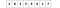The array we will be sorting with merge sort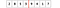Finding the mid point of the given array, either by (l+(r-l)/2) or size/2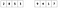Creating 2 sub-arrays of equal parts from our given array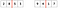Finding midpoints of sub-arrays till we reach the base caseCreating 2 sub-arrays of equal parts from our given sub-arraysFinding midpoints of sub-arrays till we reach the base case and splitting them into 2 sub-arraysAll the subarrays become of size so base-case is satisfied, merge function is calledMerge function compares adjacent sub-arrays and sorts into new sub-array.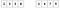Newly created and sorted sub-arrays are compared by the merge function joined together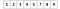Finally we a sorted array with the elements of our original array

## Taking a look at the actual code:

We will look at the code for our mergeSort function as well as our merge function.

MergeSort function:

Merge function:

Output for example array:

## Merge Sort Time and Space Complexity

→Space Complexity

Auxiliary Space: O(n)
Sorting In Place: No
Algorithm: Divide and Conquer

→ Time Complexity

Merge Sort is a recursive algorithm and time complexity can be expressed as following recurrence relation.

T(n) = 2T(n/2) + O(n)

The solution of the above recurrence is O(nLogn). The list of size N is divided into a max of Logn parts, and the merging of all sublists into a single list takes O(N) time, the worst-case run time of this algorithm is O(nLogn)

Best Case Time Complexity: O(n*log n)

Worst Case Time Complexity: O(n*log n)

Average Time Complexity: O(n*log n)

The time complexity of MergeSort is O(n*Log n) in all the 3 cases (worst, average and best) as the mergesort always divides the array into two halves and takes linear time to merge two halves.

Merge Sort is quite fast and has a time complexity of `O(n*log n)`. It is also a stable sort, which means the "equal" elements are ordered in the same order in the sorted list.

In this section, we will understand why the running time for merge sort is `O(n*log n)`.

## Pros and Cons of MergeSort:

Pros:

• It is quicker for larger lists because unlike insertion it doesn’t go through the whole list several times.
• The merge sort is slightly faster than the heap sort for larger sets
• 𝑂(𝑛𝑙𝑜𝑔𝑛) worst-case asymptotic complexity.
• Stable sorting algorithm

Cons:

• Slower comparative to the other sort algorithms for smaller data sets
• Marginally slower than quicksort in practice
• Goes through the whole process even if the list is sorted
• It uses more memory space to store the sub-elements of the initial split list.
• It requires twice the memory of the heap sort because of the second array.

## Overview:

Merge Sort is a divide and conquer algorithm. It works by recursively breaking down a problem into two or more sub-problems of the same or related type until these become simple enough to be solved directly. The solutions to the sub-problems are then combined to give a solution to the original problem.

It is one of the most well-recognised algorithms in computer science as it is quicker for larger lists because unlike insertion it doesn’t go through the whole list several times but comparatively slower for smaller data sets.

Its time complexity being O(n*log n) for all cases(best, worst, average) as it always divides the array into 2 parts and merges them and its space complexity O(n).

References: